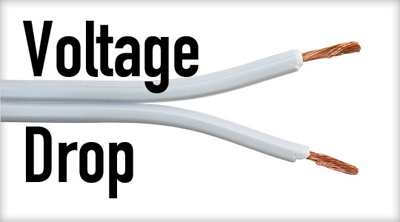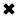# Voltage Drop Explained#### Voltage drop is the loss of voltage caused by the flow of current through a resistance.

Voltage drop (VD) occurs when the voltage at the end of a run of cable is lower than at the beginning. Any length or size of wires will have some resistance, and running a current through this dc resistance will cause the voltage to drop. As the length of the cable increases, so does its resistance and reactance increase in proportion. Hence, VD is particularly a problem with long cables runs, for example in larger buildings or on larger properties such as farms. This technique is often used when properly sizing conductors in any single phase, line to line electrical circuit. This can be measured with a voltage drop calculator.

Electrical cables carrying current always present inherent resistance, or impedance, to the flow of current. VD is measured as the amount of voltage loss which occurs through all or part of a circuit due to what is called cable "impedance" in volts.

Too much VD in a cable cross sectional area can cause lights to flicker or burn dimly, heaters to heat poorly, and motors to run hotter than normal and burn out. This condition causes the load to work harder with less voltage pushing the current.

How is this solved?

To decrease the VD in a circuit, you need to increase the size (cross section) of your conductors – this is done to lower the overall resistance of the cable length. Certainly, larger copper or aluminum cable sizes increase cost, so it’s important to calculate VD and find the optimum voltage wires size that will reduce VD to safe levels while remaining cost-effective.

How do you calculate voltage drop?

VD is the loss of voltage caused by the flow of current flow through a resistance. The greater the resistance the greater the VD To check the VD, use a voltmeter connected between the poinL where the VD is to be measured. In DC circuits and AC resistive circuits the total of all the voltage drops across series-connected loads should add up to the voltage applied to the circuit (Figure 1).

Each load device must receive its rated voltage to operate properly. If not enough voltage is available, the device will not operate as it should. You should always be certain the voltage you are going to measure does not exceed the range of the voltmeter. This may be difficult if the voltage is unknown. If such is the case, you should always start with the highest range. Attempting to measure a voltage higher than the voltmeter can handle may cause damage to the voltmeter. At times you may be required to measure a voltage from a specific point in the circuit to ground or a common reference point (Figure 8-15). To do this, first connect the black common test probe of the voltmeter to the circuit ground or common. Then connect the red test probe to whatever point in the circuit you want to measure.

To accurately calculate the VD for a given cable size, length, and current, you need to accurately know the resistance of the type of cable you’re using. However, AS3000 outlines a simplified method that can be used.

The table below is taken from AS3000 – it specifies ‘Am per %Vd‘ (amp metres per % voltage drop) for each cable size.  To calculate the VD for a circuit as a percentage, multiply the current (amps) by the cable length (metres); then divide this Ohm number by the value in the table.

For example, a 30m run of 6mm2 cable carrying 3 phase 32A will result in 1.5% drop: 32A x 30m = 960Am / 615 = 1.5%.Fig. 1. Measuring voltage drops across loads

Read our companion article Voltage Drop Calculator

##### Basic Electricity Handbook, Vol. 1• FULLY ILLUSTRATED
• GREAT FOR SCHOOL PROJECTS
• GREAT PRICE: \$5.99
• ...

This 100+ page e-book is a great guide for those who have a basic interest in the field of electricity. This well-illustrated e-book, coupled with some basic knowledge of electricity, will give you a broad theoretical background in this fundamental subject.

CONTENTS
• FUNDAMENTALS OF ELECTRICITY
• HOW DOES ELECTRICITY WORK?
• HOW IS ELECTRICITY GENERATED?
• WHERE DOES ELECTRICITY COME FROM?
• BASIC HOME WIRING
• ELECTRICITY FOR STUDENTS
• ENERGY SAVING TIPS

##### TRAINING EF COURSES

Content Community Connection
Top